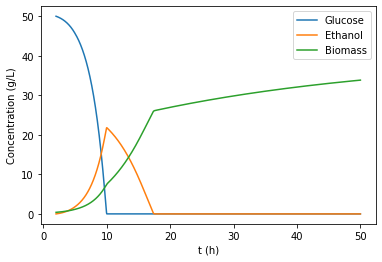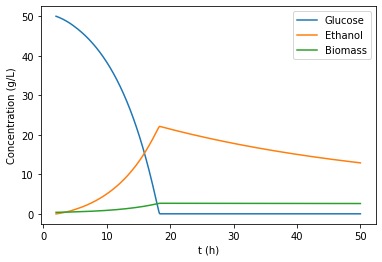Here, we are going to learn about the mathematical model of the aerobic growth of Saccharomyces cerevisiae

First, we need to create a class, as we will be using object-oriented modelling (see introductory video in biovl.com) and we initialize all the parameters in the function def init()

In :
class Scerevisiae_Aero:
def __init__(self):
self.Yox_XG = 0.8 #
self.Yred_XG = 0.05
self.Yox_XE =  0.72
self.Y_OG = 1.067
self.Y_EG = 0.5
self.Y_OE = 1.5
self.q_g = 3.5
self.q_o = 0.37
self.q_e = 0.32
self.t_lag = 4.66
self.Kg = 0.17
self.Ke = 0.56
self.Ko = 0.0001
self.Ki = 0.31
self.O_sat = 0.00755
self.kla = 1004

self.G0 = 50
self.E0 = 0.0
self.O0 = 0.00755
self.X0 = 0.4
self.V0 = 20
self.T0 = 30

self.t_end = 50
self.t_start = 2
self.steps = (self.t_end - self.t_start)*24
self.Cin = 50
self.Fconst = 0.5
self.t_feed_start = 5

self.u= 75 #mass flow of cooling water

def rxn(self, C,t):
#number of components
n = 4
m = 4

#initialize the stoichiometric matrix, s
s = np.zeros((m,n))
s[0,0] = -1
s[0,1] = 0
s[0,2] = -self.Y_OG
s[0,3] = self.Yox_XG

s[1,0] = -1
s[1,1] = self.Y_EG
s[1,2] = 0
s[1,3] = self.Yred_XG

s[2,0] = 0
s[2,1] = -1
s[2,2] = -self.Y_OE
s[2,3] = self.Yox_XE

s[3,0] = 0
s[3,1] = 0
s[3,2] = 1
s[3,3] = 0

#initialize the rate vector
rho = np.zeros((4,1))

##initialize the overall conversion vector
r=np.zeros((4,1))

#Developing the matrix, the overall conversion rate is stoichiometric *rates
rho[0,0] = ((1/self.Y_OG)*min(self.q_o*(C/(C+self.Ko)),self.Y_OG*(self.q_g*(C/(C+self.Kg)))))*C
rho[1,0] = ((1-math.exp(-t/self.t_lag))*((self.q_g*(C/(C+self.Kg)))-(1/self.Y_OG)*min(self.q_o*(C/(C+self.Ko)),self.Y_OG*(self.q_g*(C/(C+self.Kg))))))*C
rho[2,0] = ((1/self.Y_OE)*min(self.q_o*(C/(C+self.Ko))-(1/self.Y_OG)*min(self.q_o*(C/(C+self.Ko)),self.Y_OG*(self.q_g*(C/(C+self.Kg)))),self.Y_OE*(self.q_e*(C/(C+self.Ke))*(self.Ki/(C+self.Ki)))))*C
rho[3,0] = self.kla*(self.O_sat - C)

#Volume balance
if (t>=self.t_feed_start):
Fin = self.Fconst
Fout = self.Fconst
else:
Fin = 0
Fout = 0
F = Fin - Fout

#Developing the matrix, the overall conversion rate is stoichiometric *rates
r[0,0] = (s[0,0]*rho[0,0])+(s[1,0]*rho[1,0])+(s[2,0]*rho[2,0])+(s[3,0]*rho[3,0])
r[1,0] = (s[0,1]*rho[0,0])+(s[1,1]*rho[1,0])+(s[2,1]*rho[2,0])+(s[3,1]*rho[3,0])
r[2,0] = (s[0,2]*rho[0,0])+(s[1,2]*rho[1,0])+(s[2,2]*rho[2,0])+(s[3,2]*rho[3,0])
r[3,0] = (s[0,3]*rho[0,0])+(s[1,3]*rho[1,0])+(s[2,3]*rho[2,0])+(s[3,3]*rho[3,0])

#Solving the mass balances
dGdt = r[0,0] -F/C*C + Fin/C*self.Cin - Fout/C*C
dEdt = r[1,0] -F/C*C - Fout/C*C
dOdt = r[2,0]
dXdt = r[3,0] -F/C*C - Fout/C*C
dVdt = F

#Solving the heat balance
#Metabolic heat: [W]=[J/s], dhc0 from book "Bioprocess Engineering Principles" (Pauline M. Doran) : Appendix Table C.8
dHrxndt =   dXdt*C*(-21200) #[J/s]  + dGdt*C*(15580)- dEdt*C*(29710)
#Shaft work 1 W/L1
W = -1*C #[J/S] negative because exothermic
#Cooling just an initial value (constant cooling to see what happens)
#dQdt = -0.03*C*(-21200) #[J/S]
#velocity of cooling water: u [m3/h] -->controlled by PID
#Mass flow cooling water
M= self.u/3600*1000 #[kg/s]
#Define Tin = 5 C, Tout=TReactor
#heat capacity water = 4190 J/kgK
Tin = 5
#Estimate water at outlet same as Temp in reactor
Tout = C
cpc = 4190
#Calculate Q from Eq 9.47
Q=-M*cpc*(Tout-Tin) # J/s
#Calculate Temperature change
dTdt = -1*(dHrxndt - Q + W)/(C*1000*4.1868) #[K/s]

return [dGdt,dEdt,dOdt,dXdt,dVdt, dTdt]

def solve(self):
#time
t = np.linspace(self.t_start, self.t_end, self.steps)
C0 = [self.G0, self.E0, self.O0, self.X0, self.V0, self.T0]
C = odeint(self.rxn, C0, t, rtol = 1e-7, mxstep= 500000)
return C,t



Now, we do write the decomposed reactions, using a matrix notation

Once we have implemented the model, we need to solve the differential equations of [dGdt,dEdt,dOdt,dXdt,dVdt] through a solver. In this case the solver will be scipy.odeint

Function def solve(self) starts with initial concentrations (C0) and through the self.rxn (= def rxn(self, C,t)), calculate the concentration for the time step 1, then it goes back to def solve and using the concentrations for the time step 1, calculates the concentrations for the time step 2, etc.

However, for this to work, we need to import some packages

In :
#Package for solving the ODE
from scipy.integrate import odeint
#Package for plotting
import math
#Package for the use of vectors and matrix
import numpy as np
import sys
import os
import matplotlib.pyplot as plt

In :
model = Scerevisiae_Aero()
solution_model = model.solve()

In :
C = solution_model
G = C[:,0]
E = C[:,1]
X = C[:,3]

t = solution_model

In :
plt.plot(t, G, label = 'Glucose')
plt.plot(t, E,label = 'Ethanol')
plt.plot(t, X,label = 'Biomass')

plt.xlabel('t (h)')
plt.ylabel('Concentration (g/L)')
plt.legend()

plt.show

Out:
<function matplotlib.pyplot.show(*args, **kw)># How to... modify the model¶

First, we learn how we change variables and recalculate the model

In :
model = Scerevisiae_Aero()
print('Old value')
print(model.Yox_XG)

model_new = Scerevisiae_Aero()

model_new.Yox_XG = 0.02
print('New value')
print(model_new.Yox_XG)

solution_model_new = model_new.solve()

0.8
0.02

In :
C = solution_model_new
G = C[:,0]
E = C[:,1]
X = C[:,3]

t = solution_model_new

plt.plot(t, G, label = 'Glucose')
plt.plot(t, E,label = 'Ethanol')
plt.plot(t, X,label = 'Biomass')

plt.xlabel('t (h)')
plt.ylabel('Concentration (g/L)')
plt.legend()

plt.show

Out:
<function matplotlib.pyplot.show(*args, **kw)>What differences do you see?

In [ ]:



## Question for you¶

Now that you know how to change a variable and recalculate the model; propose a new value for a variable with the aim to increase the consumption of ethanol.

Plot it so you can see the effect

In [ ]: